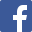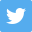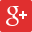1931
Senior Section P W D L F A GD GA Pt Adj Reserve Section Isthmian League `1.` `Wimbledon` `26` `17` `2` `7` `60` `35` `25` `1.71` `36` `2.` `Ilford` `26` `13` `9` `4` `71` `45` `26` `1.58` `35` `3.` `Dulwich Hamlet` `26` `15` `3` `8` `69` `43` `26` `1.60` `33` `4.` `Wycombe Wanderers` `26` `14` `5` `7` `72` `50` `22` `1.44` `33` `5.` `Oxford City` `26` `15` `2` `9` `63` `49` `14` `1.29` `32` `6.` `Kingstonian` `26` `13` `3` `10` `71` `50` `21` `1.42` `29` `7.` `Tufnell Park` `26` `9` `7` `10` `50` `48` `2` `1.04` `25` `8.` `Nunhead` `26` `9` `7` `10` `54` `61` `-7` `0.89` `25` `9.` `The Casuals` `26` `10` `4` `12` `59` `65` `-6` `0.91` `24` `10.` `Clapton` `26` `9` `5` `12` `50` `57` `-7` `0.88` `23` `11.` `Leytonstone` `26` `9` `3` `14` `36` `61` `-25` `0.59` `21` `12.` `St. Albans City` `26` `8` `4` `14` `57` `78` `-21` `0.73` `20` `13.` `Woking` `26` `6` `5` `15` `44` `64` `-20` `0.69` `17` `14.` `London Caledonians` `26` `2` `7` `17` `24` `74` `-50` `0.32` `11` ` ` `364` `149` `66` `149` `780` `780` `0` `364` `0` Isthmian League `1.` `Dulwich Hamlet (reserves)` `26` `17` `2` `7` `124` `48` `76` `2.58` `36` `2.` `The Casuals (reserves)` `26` `16` `2` `8` `84` `67` `17` `1.25` `34` `3.` `Wimbledon (reserves)` `26` `16` `0` `10` `96` `62` `34` `1.55` `32` `4.` `Leytonstone (reserves)` `26` `14` `3` `9` `70` `50` `20` `1.40` `31` `5.` `Kingstonian (reserves)` `26` `13` `4` `9` `66` `63` `3` `1.05` `30` `6.` `Tufnell Park (reserves)` `26` `12` `1` `13` `60` `65` `-5` `0.92` `25` `7.` `London Caledonians (reserves)` `26` `10` `4` `12` `67` `74` `-7` `0.91` `24` `8.` `Oxford City (reserves)` `26` `10` `4` `12` `73` `90` `-17` `0.81` `24` `9.` `St. Albans City (reserves)` `26` `11` `1` `14` `71` `75` `-4` `0.95` `23` `10.` `Wycombe Wanderers (reserves)` `26` `8` `6` `12` `71` `77` `-6` `0.92` `22` `11.` `Ilford (reserves)` `26` `10` `2` `14` `65` `78` `-13` `0.83` `22` `12.` `Woking (reserves)` `26` `10` `2` `14` `51` `85` `-34` `0.60` `22` `13.` `Clapton (reserves)` `26` `8` `4` `14` `48` `77` `-29` `0.62` `20` `14.` `Nunhead (reserves)` `26` `7` `5` `14` `47` `82` `-35` `0.57` `19` ` ` `364` `162` `40` `162` `993` `993` `0` `364` `0`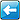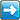This page has been visited 774 times. The contents of this site are (c) their respective owners. If you wish to use anything within, please check their status first. If you have any information, photographs, comments, additions, memorabilia or corrections to this site please contact us